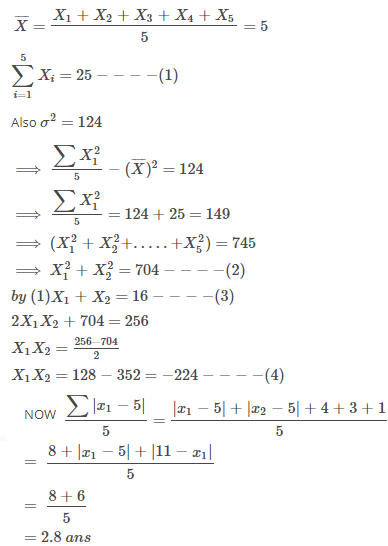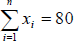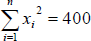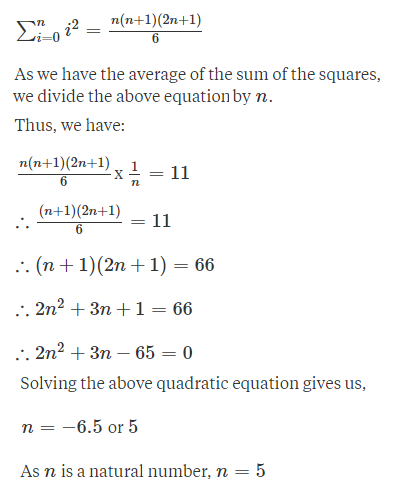## Aspire's Library

A Place for Latest Exam wise Questions, Videos, Previous Year Papers,
Study Stuff for MCA Examinations

## NIMCET Previous Year Questions (PYQs)

#### NIMCET Statistics Measures Of Central Tendency PYQ

NIMCET PYQ
The mean of 5 observation is 5 and their variance is 12.4. If three of the observations are 1,2 and 6; then the mean deviation from the mean of the data is:

NIMCET Previous Year PYQNIMCET NIMCET 2019 PYQ

#### SolutionNIMCET PYQ
If the mean deviation 1, 1+d, 1+2d, … , 1+100d from their mean is 255, then d is equal to

NIMCET Previous Year PYQNIMCET NIMCET 2019 PYQ

#### Solution

NIMCET PYQ
Ifand, then a possible value of n is among the following is

NIMCET Previous Year PYQNIMCET NIMCET 2019 PYQ

#### Solution

NIMCET PYQ

Not Available

NIMCET Previous Year PYQNIMCET NIMCET 2019 PYQ

#### Solution

NIMCET PYQ
The 10th and 50th percentiles of the observation 32, 49, 23, 29, 118 respectively are

NIMCET Previous Year PYQNIMCET NIMCET 2022 PYQ

#### Solution

NIMCET PYQ
The first three moments of a distribution about 2 are 1, 16, -40 respectively. The mean and variance of the distribution are

NIMCET Previous Year PYQNIMCET NIMCET 2022 PYQ

#### Solution

NIMCET PYQ
If ${{a}}_1,{{a}}_2,\ldots,{{a}}_n$ are any real numbers and $n$ is any positive integer, then

NIMCET Previous Year PYQNIMCET NIMCET 2022 PYQ

#### Solution

NIMCET PYQ
The mean of 25 observations was found to be 38. It was later discovered that 23 and 38 were misread as 25 and 36, then the mean is

NIMCET Previous Year PYQNIMCET NIMCET 2022 PYQ

#### Solution

NIMCET PYQ
If the mean of the squares of first n natural numbers be 11, then n is equal to?

NIMCET Previous Year PYQNIMCET NIMCET 2018 PYQ

#### SolutionNIMCET PYQ
The standard deviation of 20 numbers is 30. If each of the numbers is increased by 4, then the new standard deviation will be

NIMCET Previous Year PYQNIMCET NIMCET 2021 PYQ

#### Solution

NIMCET PYQ
If a variable takes values 0, 1, 2,…, 50 with frequencies $1,\, {{50}}_{{{C}}_1},{{50}}_{{{C}}_2},\ldots..,{{50}}_{{{C}}_{50}}$, then the AM is

NIMCET Previous Year PYQNIMCET NIMCET 2021 PYQ

#### Solution

NIMCET PYQ
Consider the following frequency distribution table.
 Class Interval 10-20 20-30 30-40 40-50 50-60 60-70 70-80 Frequency 180 $f_1$ 34 180 136 $f_2$ 50
If the total frequency is 686 and the median is 42.6, then the value of $f_1$;and $f_2$ are

NIMCET Previous Year PYQNIMCET NIMCET 2021 PYQ

#### Solution

NIMCET PYQ
A, B, C are three sets of values of x:
A: 2,3,7,1,3,2,3
B: 7,5,9,12,5,3,8
C: 4,4,11,7,2,3,4
Select the correct statement among the following:

NIMCET Previous Year PYQNIMCET NIMCET 2020 PYQ

#### Solution

A: 2, 3, 7, 1, 3, 2, 3
Increasing Order : A: 1, 2, 2, 3, 3, 3, 7
Mode = 3 (occurs maximum number of times)
Median = 3 (the middle term)

Mean =$\frac{(1+2+2+3+3+3+7)}{7}$
$=\frac{21}{7} = 3$

Hence. Mean=Median=Mode

NIMCET PYQ
Standard deviation for the following distribution is
 Size of item 6 7 8 9 10 11 12 Frequency 3 6 9 13 8 5 4

NIMCET Previous Year PYQNIMCET NIMCET 2020 PYQ

#### Solution

Total number of items in the distribution = Σ fi = 3 + 6 + 9 + 13 + 8 + 5 + 4 = 48.

The Mean (x̅) of the given set = $\rm \dfrac{\sum f_i x_i}{\sum f_i}$.

⇒ x̅ = $\rm \dfrac{6\times3+7\times6+8\times9+9\times13+10\times8+11\times5+12\times4}{48}=\dfrac{432}{48}$ = 9.

Let's calculate the variance using the formula: $\rm \sigma^2 =\dfrac{\sum x_i^2}{n}-\bar x^2$.

$\rm \dfrac{\sum {x_i}^2}{n}=\dfrac{6^2\times3+7^2\times6+8^2\times9+9^2\times13+10^2\times8+11^2\times5+12^2\times4}{48}=\dfrac{4012}{48}$ = 83.58.

∴ σ2 = 83.58 - 92 = 83.58 - 81 = 2.58.

And, Standard Deviation (σ) = $\rm \sqrt{\sigma^2}=\sqrt{Variance}=\sqrt{2.58}$ ≈ 1.607.

NIMCET PYQ
The mean of 5 observation is 5 and their variance is 124. If three of the observations are 1,2 and 6; then the mean deviation from the mean of the data is:

NIMCET Previous Year PYQNIMCET NIMCET 2023 PYQ

#### Solution

NIMCET PYQ
For a group of 100 candidates, the mean and standard deviation of scores were found to be 40 and 15 respectively. Later on, it was found that the scores 25 and 35 were misread as 52 and 53 respectively. Then the corrected mean and standard deviation corresponding to the corrected figures are

NIMCET Previous Year PYQNIMCET NIMCET 2023 PYQ

#### Solution

NIMCET PYQ
Consider the following frequency distribution table.
 Class interval 10-20 20-30 30-40 40-50 50-60 60-70 70-80 Frequency 180 $f_1$ 34 180 136 $f_2$ 50

If the total frequency is 685 & median is 42.6 then the values of $f_1$  and $f_2$  are

NIMCET Previous Year PYQNIMCET NIMCET 2023 PYQ

#### NIMCET

Online Test Series,
and More.

#### NIMCET

Online Test Series,